GFG App
Open AppBrowser
Continue

# Implement Quicksort with first element as pivot

QuickSort is a Divide and Conquer algorithm. It picks an element as a pivot and partitions the given array around the pivot. There are many different versions of quickSort that pick the pivot in different ways.

• Always pick the first element as a pivot.
• Always pick the last element as a pivot.
• Pick a random element as a pivot.
• Pick the median as the pivot.

Note: Here we will be implementing quick sort by picking the first element as the pivot.

## Quick Sort by picking the first element as the Pivot.

The key function in quick sort is a partition. The target of partitions is to put the pivot in its correct position if the array is sorted and the smaller (or equal) to its left and higher elements to its right and do all this in linear time.

### Partition Algorithm:

There can be many ways to do partition, the following pseudo-code adopts the method given in the CLRS book.

• We start from the leftmost element and keep track of the index of smaller (or equal) elements as i
• While traversing, if we find a smaller (or equal) element, we swap the current element with arr[i].
• Otherwise, we ignore the current element.

### Pseudo Code for recursive QuickSort function:

// low  –> Starting index,  high  –> Ending index

quickSort(arr[], low, high) {
if (low < high) {

// pi is partitioning index, arr[pi] is now at right place
pi = partition(arr, low, high);
quickSort(arr, low, pi – 1);  // Before pi
quickSort(arr, pi + 1, high); // After pi
}
}

### Pseudo code for partition() function

/* This function takes first element as pivot, places the pivot element at its correct position in sorted array, and places all smaller (smaller than or equal to pivot) to left of pivot and all greater elements to right of pivot */

partition (arr[], low, high) {
// first element as pivot
pivot = arr[low]
k = high
for (i = high; i > low; i–) {
if (arr[i] > pivot){
swap arr[i] and arr[k];
k–;
}
}
swap arr[k] and arr[low]
return k-1;
}

## Illustration of partition() :

Consider: arr[] = { 7,   6,   10,   5,   9,   2,   1,   15,   7 }

First Partition: low = 0, high = 8, pivot = arr[low] = 7
Initialize index of right most element k = high = 8.

• Traverse from i = high to low:
• if arr[i] is greater than pivot:
• Swap arr[i] and arr[k].
• Decrement k;
• At the end swap arr[low] and arr[k].

Now the correct position of pivot is index 5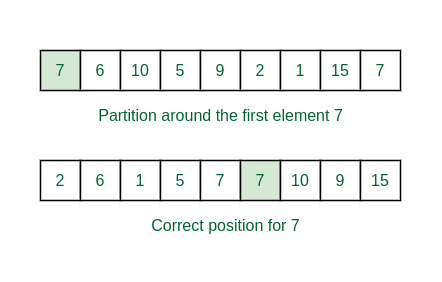First partition

Second Partition: low = 0, high = 4, pivot = arr[low] = 2
Similarly initialize k = high = 4;

The correct position of 2 becomes index 1. And the left part is only one element and the right part has {6, 5, 7}.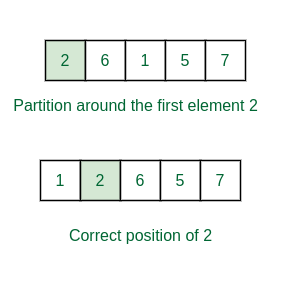Partition of the left half

On the other hand partition happens on the segment [6, 8] i.e., the array {10, 9, 15}.
Here low = 6, high = 8, pivot = 10 and k = 8.

The correct position of 10 becomes index 7 and the right and left part both has only one element.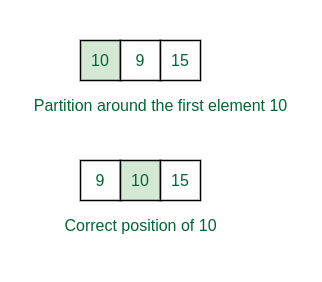Partition of the right half

Third partition:  Here partition the segment {6, 5, 7}. The low = 2, high = 4, pivot = 6 and k = 4.
If the same process is applied, we get correct position of 6 as index 3 and the left and the right part is having only one element.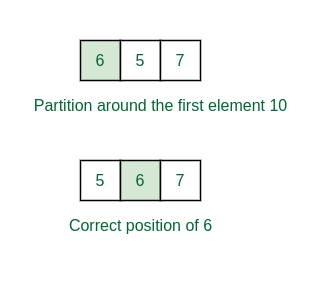Third partition

The total array becomes sorted in this way. Check the below image for the recursion tree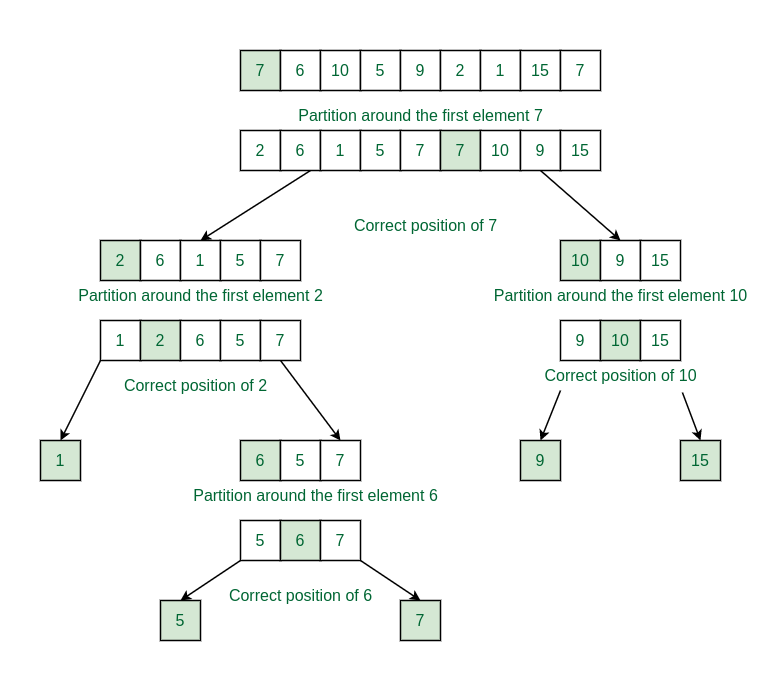Recursion tree for partitions

Follow the below steps to implement the approach.

• Use a recursive function (say quickSort) to initialize the function.
• Call the partition function to partition the array and inside the partition function do the following
• Take the first element as pivot and initialize and iterator k = high.
• Iterate in a for loop from i = high to low+1:
• If arr[i] is greater than pivot then swap arr[i] and arr[k] and decrement k.
• After the iteration is swap the pivot with arr[k].
• Return k-1 as the point of partition.
• Now recursively call quickSort for the left half and right half of the partition index.

Implementation of the above approach.

## C++

 `/* C++ implementation of QuickSort by taking first element` ` ``* as pivot */` `#include ` `using` `namespace` `std;`   `/*This function takes first element as pivot, the function` `places the pivot element(first element) on its sorted` `position and all the element lesser than pivot will placed` `left to it, and all the element greater than pivot placed` `right to it.*/`   `int` `partition(``int` `arr[], ``int` `low, ``int` `high)` `{`   `    ``// First element as pivot` `    ``int` `pivot = arr[low];` `    ``int` `st = low; ``// st points to the starting of array` `    ``int` `end = high; ``// end points to the ending of the array` `    ``int` `k = high;` `    ``for` `(``int` `i = high; i > low; i--) {` `        ``if` `(arr[i] > pivot)` `            ``swap(arr[i], arr[k--]);` `    ``}` `    ``swap(arr[low], arr[k]);` `    ``// As we got pivot element index is end` `    ``// now pivot element is at its sorted position` `    ``// return pivot element index (end)` `    ``return` `k;` `}`   `/* The main function that implements QuickSort` `arr[] --> Array to be sorted,` `low --> Starting index,` `high --> Ending index */` `void` `quickSort(``int` `arr[], ``int` `low, ``int` `high)` `{` `    ``// If low is lesser than high` `    ``if` `(low < high) {` `        ``// idx is index of pivot element which is at its` `        ``// sorted position` `        ``int` `idx = partition(arr, low, high);`   `        ``// Separately sort elements before` `        ``// partition and after partition` `        ``quickSort(arr, low, idx - 1);` `        ``quickSort(arr, idx + 1, high);` `    ``}` `}`   `/* Function to print an array */` `void` `printArray(``int` `arr[], ``int` `size)` `{` `    ``int` `i;` `    ``for` `(i = 0; i < size; i++)` `        ``cout << arr[i] << ``" "``;` `    ``cout << endl;` `}`   `// Driver Code` `int` `main()` `{` `    ``int` `arr[] = { 7, 6, 10, 5, 9, 2, 1, 15, 7 };` `    ``int` `n = ``sizeof``(arr) / ``sizeof``(arr);` `    ``quickSort(arr, 0, n - 1);` `    ``cout << ``"Sorted array: \n"``;` `    ``printArray(arr, n);` `    ``return` `0;` `}`   `// This Code is contributed by Harsh Raghav`

## Python3

 `# This function takes first element as pivot, the function` `# places the pivot element(first element) on its sorted` `# position and all the element lesser than pivot will placed` `# left to it, and all the element greater than pivot placed` `# right to it.` `def` `partition(array, low, high):` `  `  `    ``# First Element as pivot` `    ``pivot ``=` `array[low]` `    `  `    ``# st points to the starting of array` `    ``start ``=` `low ``+` `1` `    `  `    ``# end points to the ending of the array` `    ``end ``=` `high`   `    ``while` `True``:` `        ``# It indicates we have already moved all the elements to their correct side of the pivot` `        ``while` `start <``=` `end ``and` `array[end] >``=` `pivot:` `            ``end ``=` `end ``-` `1`   `        ``# Opposite process` `        ``while` `start <``=` `end ``and` `array[start] <``=` `pivot:` `            ``start ``=` `start ``+` `1`   `        ``# Case in which we will exit the loop` `        ``if` `start <``=` `end:` `            ``array[start], array[end] ``=` `array[end], array[start]` `            ``# The loop continues` `        ``else``:` `            ``# We exit out of the loop` `            ``break`   `    ``array[low], array[end] ``=` `array[end], array[low]` `    ``# As we got pivot element index is end` `    ``# now pivot element is at its sorted position` `    ``# return pivot element index (end)` `    ``return` `end`   `# The main function that implements QuickSort` `# arr[] --> Array to be sorted,` `# low --> Starting index,` `# high --> Ending index` `def` `quick_sort(array, start, end):`   `    ``# If low is lesser than high` `    ``if` `start < end:` `      `  `        ``# idx is index of pivot element which is at its` `        ``# sorted position` `        ``idx ``=` `partition(array, start, end)` `        `  `        ``# Separately sort elements before` `        ``# partition and after partition` `        ``quick_sort(array, start, idx``-``1``)` `        ``quick_sort(array, idx``+``1``, end)`   `# Function to print an array` `def` `print_arr(arr, n):` `    ``for` `i ``in` `range``(n):` `        ``print``(arr[i], end``=``" "``)` `    ``print``()`   `# Driver Code` `arr1 ``=` `[``7``, ``6``, ``10``, ``5``, ``9``, ``2``, ``1``, ``15``, ``7``]` `quick_sort(arr1, ``0``, ``len``(arr1)``-``1``)` `print_arr(arr1, ``len``(arr1))`   `# This code is contributed by Aditya Sharma`

## Java

 `// Java implementation of QuickSort by taking first element` `// as pivot` `import` `java.util.Arrays;`   `class` `QuickSort {` `    ``/* This function takes first element as pivot, the` `    ``function places the pivot element(first element) on its` `    ``sorted position and all the element lesser than pivot` `    ``will placed left to it, and all the element greater than` `    ``pivot placed right to it.*/` `    ``int` `partition(``int` `arr[], ``int` `low, ``int` `high)` `    ``{` `        ``// First element as pivot` `        ``int` `pivot = arr[low];` `        ``int` `st = low; ``// st points to the starting of array` `        ``int` `end` `            ``= high; ``// end points to the ending of the array` `        ``int` `k = high;` `        ``for` `(``int` `i = high; i > low; i--) {` `            ``if` `(arr[i] > pivot)` `                ``swap(arr, i, k--);` `        ``}` `        ``swap(arr, low, k);` `        ``// As we got pivot element index is end` `        ``// now pivot element is at its sorted position` `        ``// return pivot element index (end)` `        ``return` `k;` `    ``}`   `    ``// Function to swap two elements` `    ``public` `static` `void` `swap(``int``[] arr, ``int` `i, ``int` `j)` `    ``{` `        ``int` `temp = arr[i];` `        ``arr[i] = arr[j];` `        ``arr[j] = temp;` `    ``}`   `    ``/* The main function that implements QuickSort` `    ``arr[] --> Array to be sorted,` `    ``low --> Starting index,` `    ``high --> Ending index */` `    ``void` `quickSort(``int` `arr[], ``int` `low, ``int` `high)` `    ``{` `        ``// If low is lesser than high` `        ``if` `(low < high) {` `            ``// idx is index of pivot element which is at its` `            ``// sorted position` `            ``int` `idx = partition(arr, low, high);`   `            ``// Separately sort elements before` `            ``// partition and after partition` `            ``quickSort(arr, low, idx - ``1``);` `            ``quickSort(arr, idx + ``1``, high);` `        ``}` `    ``}`   `    ``/* Function to print an array */` `    ``void` `printArray(``int` `arr[], ``int` `size)` `    ``{` `        ``int` `i;` `        ``for` `(i = ``0``; i < size; i++)` `            ``System.out.print(arr[i] + ``" "``);` `        ``System.out.println();` `    ``}`   `    ``// Driver Code` `    ``public` `static` `void` `main(String args[])` `    ``{` `        ``int` `arr[] = { ``7``, ``6``, ``10``, ``5``, ``9``, ``2``, ``1``, ``15``, ``7` `};` `        ``int` `n = arr.length;`   `        ``QuickSort ob = ``new` `QuickSort();` `        ``ob.quickSort(arr, ``0``, n - ``1``);`   `        ``System.out.println(``"Sorted array"``);` `        ``ob.printArray(arr, n);` `    ``}` `}`

## Javascript

 `function` `partition(``function` `partition(array, low, high) {` `  ``// First Element as pivot` `  ``let pivot = array[low];`   `  ``// st points to the starting of array` `  ``let start = low + 1;`   `  ``// end points to the ending of the array` `  ``let end = high;`   `  ``while` `(``true``) {` `    ``// It indicates we have already moved all the elements to their correct side of the pivot` `    ``while` `(start <= end && array[end] >= pivot) {` `      ``end--;` `    ``}`   `    ``// Opposite process` `    ``while` `(start <= end && array[start] <= pivot) {` `      ``start++;` `    ``}`   `    ``// Case in which we will exit the loop` `    ``if` `(start <= end) {` `      ``[array[start], array[end]] = [array[end], array[start]];` `      ``// The loop continues` `    ``} ``else` `{` `      ``// We exit out of the loop` `      ``break``;` `    ``}` `  ``}`   `  ``[array[low], array[end]] = [array[end], array[low]];` `  ``// As we got pivot element index is end` `  ``// now pivot element is at its sorted position` `  ``// return pivot element index (end)` `  ``return` `end;` `}`   `function` `quick_sort(array, start, end) {` `  ``// If low is lesser than high` `  ``if` `(start < end) {` `    ``// idx is index of pivot element which is at its` `    ``// sorted position` `    ``let idx = partition(array, start, end);`   `    ``// Separately sort elements before` `    ``// partition and after partition` `    ``quick_sort(array, start, idx - 1);` `    ``quick_sort(array, idx + 1, end);` `  ``}` `}`   `function` `print_arr(arr) {` `  ``console.log(arr.join(``" "``));` `}`   `// Driver Code` `let arr1 = [7, 6, 10, 5, 9, 2, 1, 15, 7];` `quick_sort(arr1, 0, arr1.length - 1);` `console.log(``"Sorted array: "``);` `print_arr(arr1);`     `//contributed by Aditya Sharma`

## C#

 `using` `System;`   `class` `QuickSort` `{` `    ``/* This function takes first element as pivot, the` `    ``function places the pivot element(first element) on its` `    ``sorted position and all the element lesser than pivot` `    ``will placed left to it, and all the element greater than` `    ``pivot placed right to it.*/` `    ``int` `partition(``int``[] arr, ``int` `low, ``int` `high)` `    ``{` `        ``// First element as pivot` `        ``int` `pivot = arr[low];` `        ``int` `st = low; ``// st points to the starting of array` `        ``int` `end = high; ``// end points to the ending of the array` `        ``int` `k = high;` `        ``for` `(``int` `i = high; i > low; i--)` `        ``{` `            ``if` `(arr[i] > pivot)` `            ``{` `                ``swap(arr, i, k--);` `            ``}` `        ``}` `        ``swap(arr, low, k);` `        ``// As we got pivot element index is end` `        ``// now pivot element is at its sorted position` `        ``// return pivot element index (end)` `        ``return` `k;` `    ``}`   `    ``// Function to swap two elements` `    ``public` `static` `void` `swap(``int``[] arr, ``int` `i, ``int` `j)` `    ``{` `        ``int` `temp = arr[i];` `        ``arr[i] = arr[j];` `        ``arr[j] = temp;` `    ``}`   `    ``/* The main function that implements QuickSort` `    ``arr[] --> Array to be sorted,` `    ``low --> Starting index,` `    ``high --> Ending index */` `    ``void` `quickSort(``int``[] arr, ``int` `low, ``int` `high)` `    ``{` `        ``// If low is lesser than high` `        ``if` `(low < high)` `        ``{` `            ``// idx is index of pivot element which is at its` `            ``// sorted position` `            ``int` `idx = partition(arr, low, high);`   `            ``// Separately sort elements before` `            ``// partition and after partition` `            ``quickSort(arr, low, idx - 1);` `            ``quickSort(arr, idx + 1, high);` `        ``}` `    ``}`   `    ``/* Function to print an array */` `    ``void` `printArray(``int``[] arr, ``int` `size)` `    ``{` `        ``int` `i;` `        ``for` `(i = 0; i < size; i++)` `            ``Console.Write(arr[i] + ``" "``);` `        ``Console.WriteLine();` `    ``}`   `    ``// Driver Code` `    ``public` `static` `void` `Main()` `    ``{` `        ``int``[] arr = { 7, 6, 10, 5, 9, 2, 1, 15, 7 };` `        ``int` `n = arr.Length;`   `        ``QuickSort ob = ``new` `QuickSort();` `        ``ob.quickSort(arr, 0, n - 1);`   `        ``Console.WriteLine(``"Sorted array"``);` `        ``ob.printArray(arr, n);` `    ``}` `}`

Output

```Sorted array:
1 2 5 6 7 7 9 10 15 ```

Complexity Analysis:

• Time Complexity:
• Average Case: O(N * logN), where N is the length of the array.
• Best Case: O(N * logN)
• Worst Case: O(N2)
• Auxiliary Space: O(1)

My Personal Notes arrow_drop_up